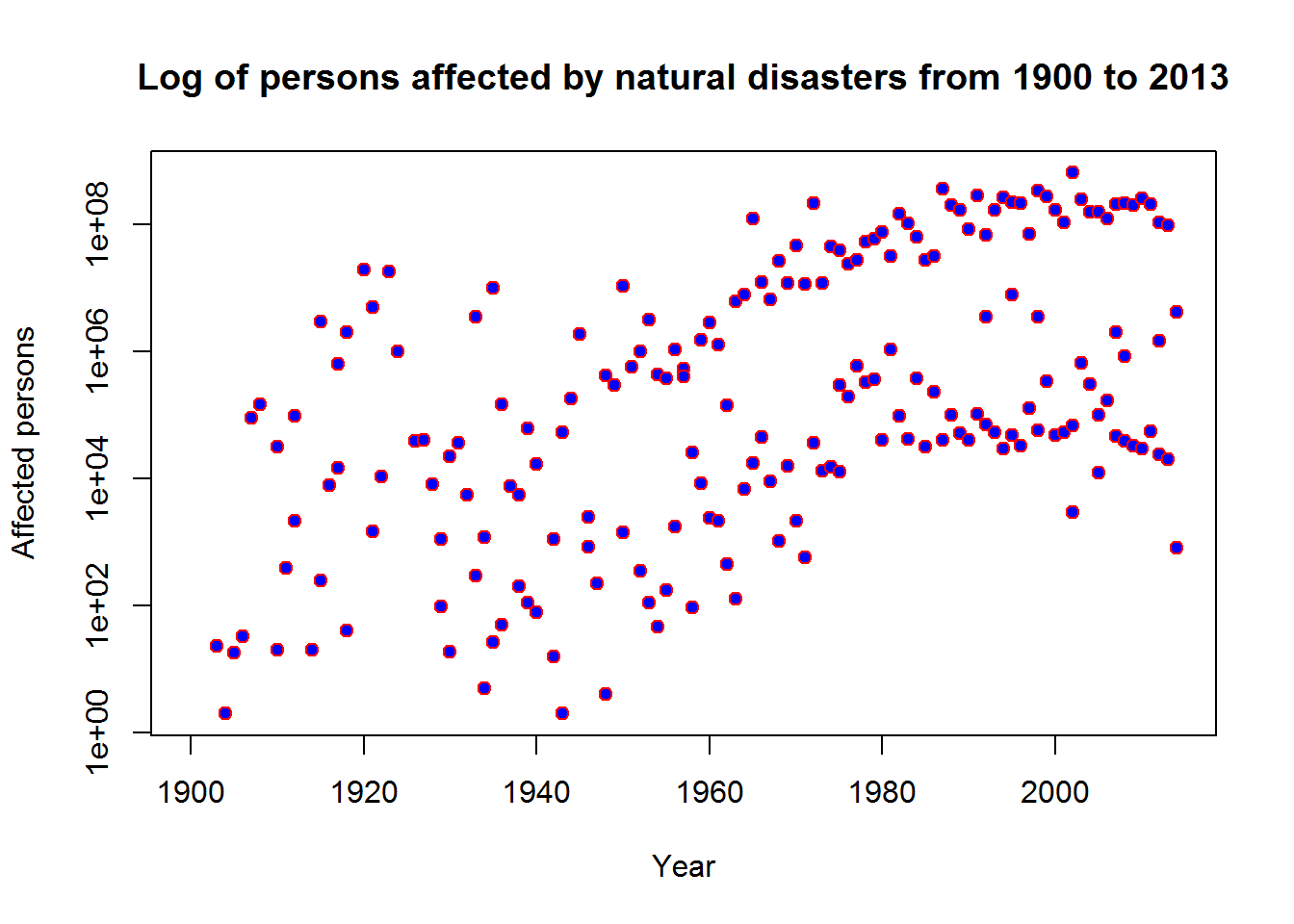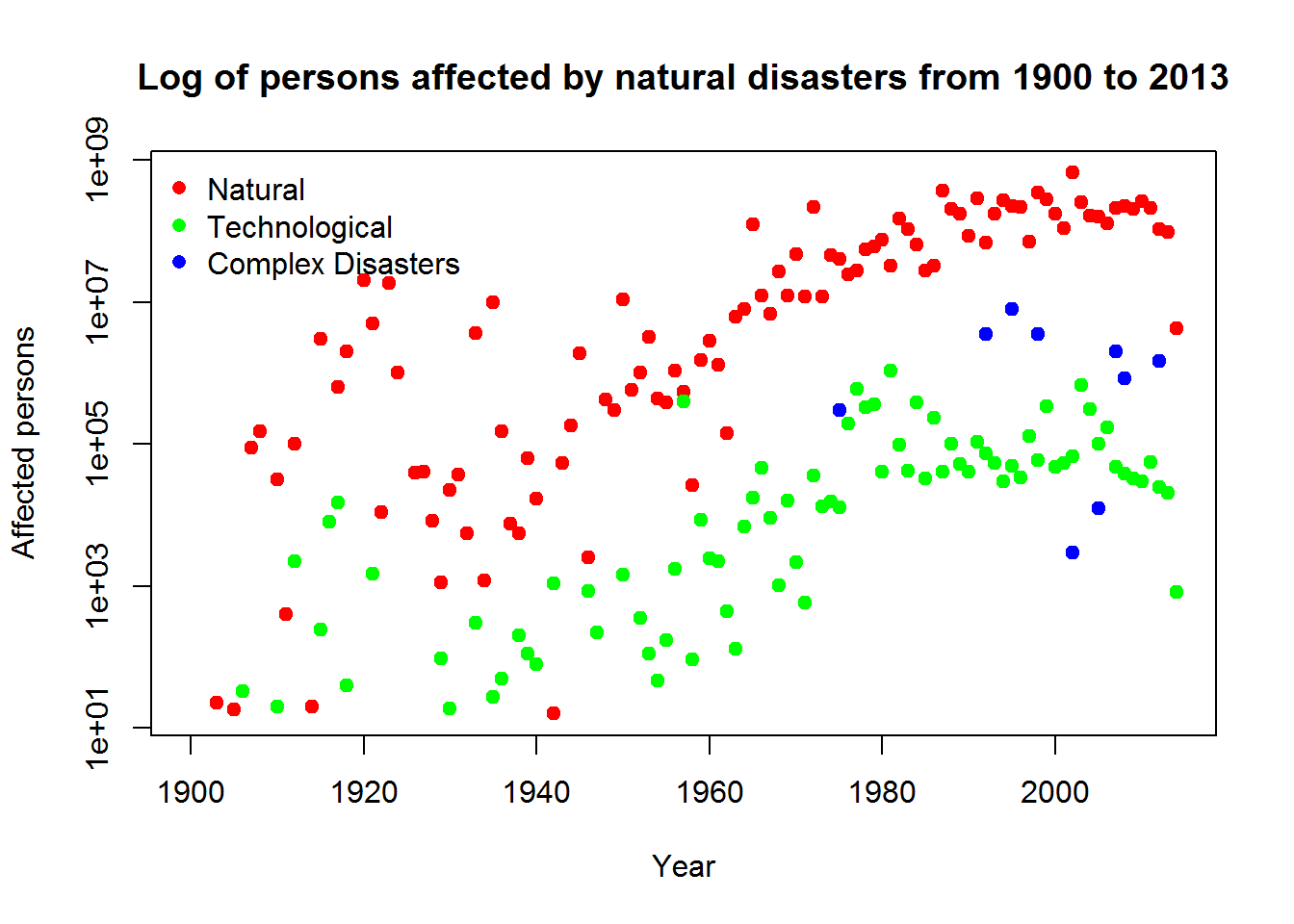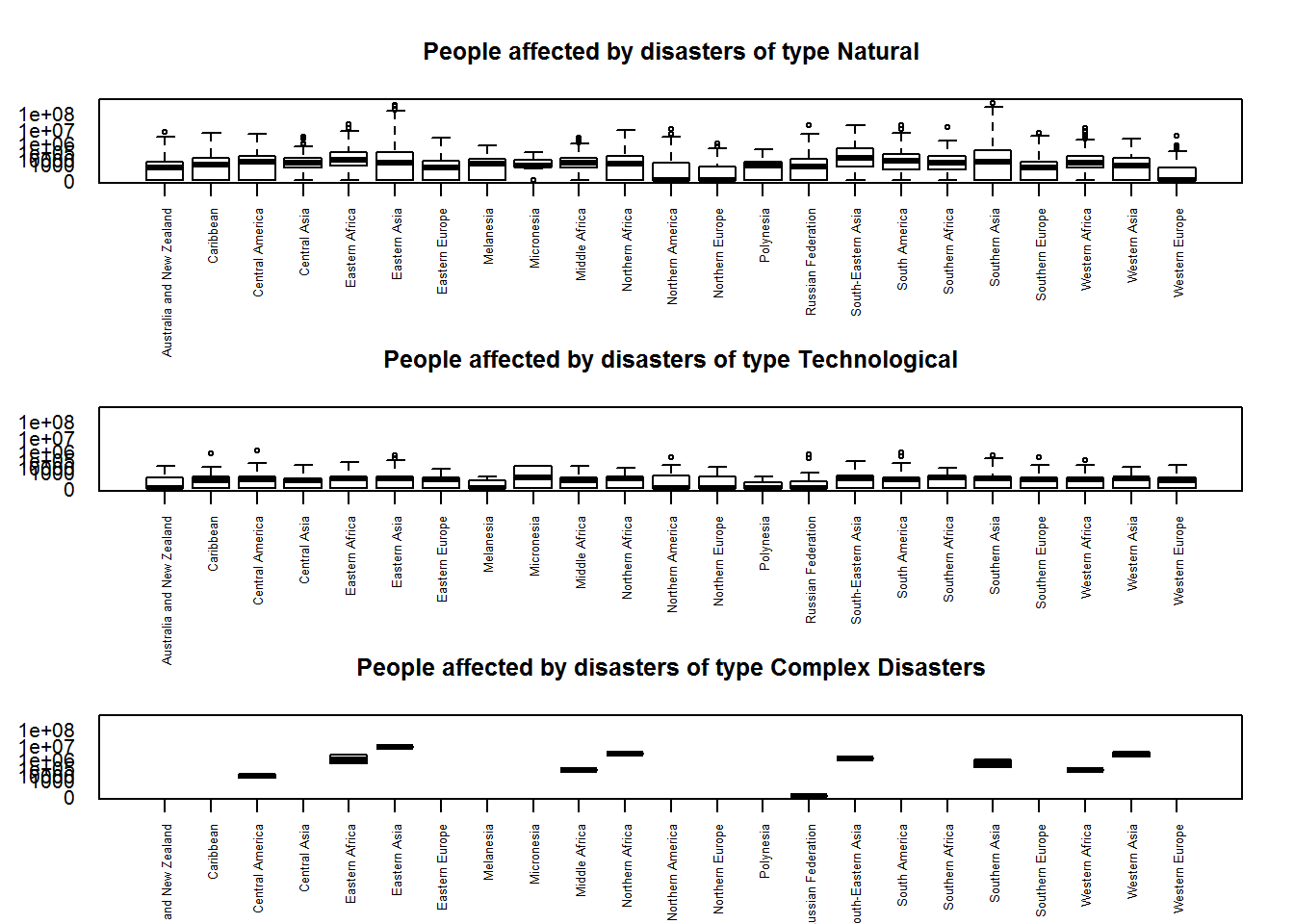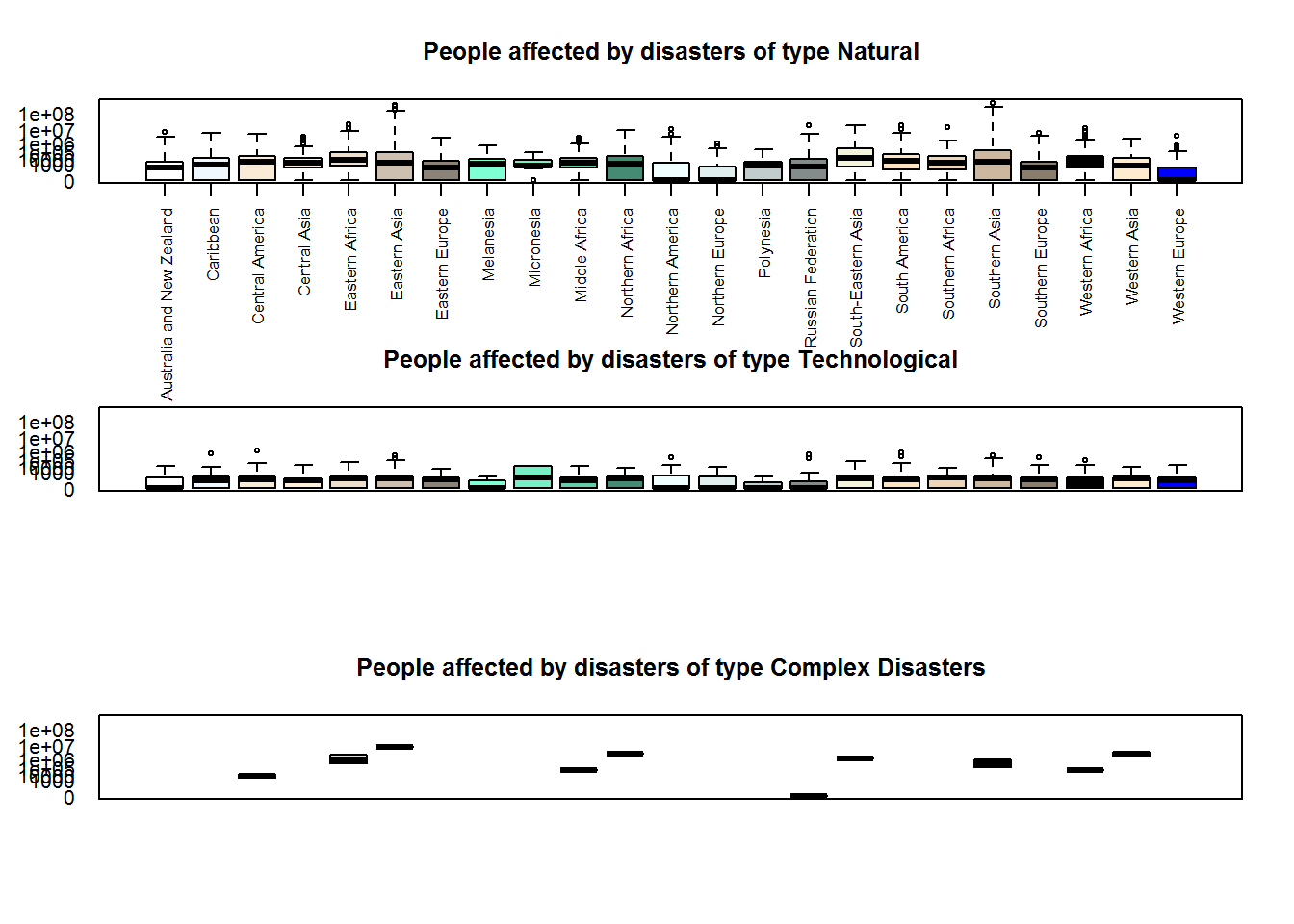BIS-Fogo

Site Tools

en:learning:schools:s01:code-examples:ba-ce-09-03

C09-3 - Multiple generic plots

The following plotting examples will revisit R’s generic plotting functions and pimp them up a little bit.

The underlaying example data is taken from our combined natural disaster and Ebola data set which is loaded in the background into the data frame called df. We will generally not interpret the plots but just look at formal aspects.

Multiple plots in one

In the following example, we will again visualize the number of totally affected people against the time line. The aggregation looks like this:

dfa <- aggregate(df$total_affected, by = list(df$disaster.group, df$year), FUN = sum) colnames(dfa) <- c("disaster.group", "year", "total_affected") In our previous example of this plot it looked like this: plot(dfa$year,
dfa$total_affected, xlab = "Year", ylab = "Affected persons", main = "Log of persons affected by natural disasters from 1900 to 2013", col = "red", bg = "blue", pch=21, log = "y") ## Warning: 39 y values <= 0 omitted from logarithmic plotLet’s plot the same data but this time using different colors for the different desaster groups. To do so, we first define a variable containint the individual disaster group values: groups <- unique(dfa$disaster.group)
groups
##  Natural           Technological     Complex Disasters
## Levels: Complex Disasters Natural Technological

As one can see, we have three different groups. Hence, we need three different colors which we define now:

colors <- c("red", "green", "blue")

As mentioned above, we have to produce the plot in one code block because of the R Markdown restrictions. We start with plotting only the values of the first group. This is done by subsetting our data frame to values of the disaster.group variable equal to the first value in the groups variable (i.e. “Natural”). As color for the symbols we use the first color in the colors variable. Hence, so far there is nothing unusal or new in this procedure.

The second and third group is then added to this first plot by using the points function instead of the plot function. While the latter draws a new plot, the former adds a plot to the last drawn plot. We do that in a for loop to save some typing.

plot(dfa$year[dfa$disaster.group == groups],
dfa$total_affected[dfa$disaster.group == groups],
xlab = "Year", ylab = "Affected persons",
main = "Log of persons affected by natural disasters from 1900 to 2013",
col = colors, bg = colors, pch=21, log = "y")
## Warning: 12 y values <= 0 omitted from logarithmic plot
for(i in seq(2, length(groups))){
points(dfa$year[dfa$disaster.group == groups[i]],
dfa$total_affected[dfa$disaster.group == groups[i]],
xlab = "Year", ylab = "Affected persons",
main = "Log of persons affected by natural disasters from 1900 to 2013",
col = colors[i], bg = colors[i], pch=21)
}

legend("topleft", pch=16, col=colors, legend=groups, bty = "n")After the complete plot has been computed, we add a legend using the legend function. The first argument places it in the top left corner, the bty argument surpresses a black box around the legend if it is set to “n”. All other arguments have the same meaning as if they would have been used within the plot function.

Multiple plots on one page

So far we had one plot per page. If you want more, just use the mfrow argument of the par function which controls a large amount of plotting parameters in the background of each generic plotting function. The first argument of the function specifies the number of rows, the second the number of columns. In the example below we use three rows (i.e. the number of disaster groups) which is equal to the length of our groups vector just created above and 1 columns. A plot will subsequently be drawn within each cell of this geometry.

In the following example we will plot three box whisker plots below each other using a simple for loop. Please note that the plotting function in this example always stays the same since we are always creating a new plot (and do not add something to a previous one). The only difference is that this time we have divided our plot page into three subsets. S

ince we want to plot square root transformed values for a better distribution along the y axis, we store the transformed values in our data frame, first:

df$total_affected_sqrt3 <- df$total_affected^0.5^3
par(mfrow=c(length(groups),1))
for(i in seq(length(groups))){
boxplot(total_affected_sqrt3 ~ region,
data = df[df$disaster.group == groups [i],], ylim = c(0, max(df$total_affected_sqrt3)), yaxt="n",
main = paste0("People affected by disasters of type ", groups[i]),
las=2, cex.axis=0.6)

ylabls <- c(0, 1000, 10000, 100000, 1000000, 10000000, 100000000)
ytics <- ylabls^(0.5)^3
axis(2, at=ytics,labels=ylabls, las=2, tck=-.01)
}Since the resulting page might look a little overloaded, we could try to lable only one of the three plots’ x axis and use colors to identify the respective boxes.

clrs <- colors(1)[1:length(unique(df$region))] par(mfrow=c(length(groups),1)) boxplot(total_affected_sqrt3 ~ region, data = df[df$disaster.group == groups ,],
ylim = c(0, max(df$total_affected_sqrt3)), yaxt="n", main = paste0("People affected by disasters of type ", groups), las=2, cex.axis=0.8, col = clrs) ylabls <- c(0, 1000, 10000, 100000, 1000000, 10000000, 100000000) ytics <- ylabls^(0.5)^3 axis(2, at=ytics,labels=ylabls, las=1, tck=-.01) for(i in seq(2, length(groups))){ boxplot(total_affected_sqrt3 ~ region, data = df[df$disaster.group == groups [i],],
ylim = c(0, max(df\$total_affected_sqrt3)), yaxt="n",
main = paste0("People affected by disasters of type ", groups[i]),
xaxt="n", col = clrs)

ylabls <- c(0, 1000, 10000, 100000, 1000000, 10000000, 100000000)
ytics <- ylabls^(0.5)^3
axis(2, at=ytics,labels=ylabls, las=2, tck=-.01)
}